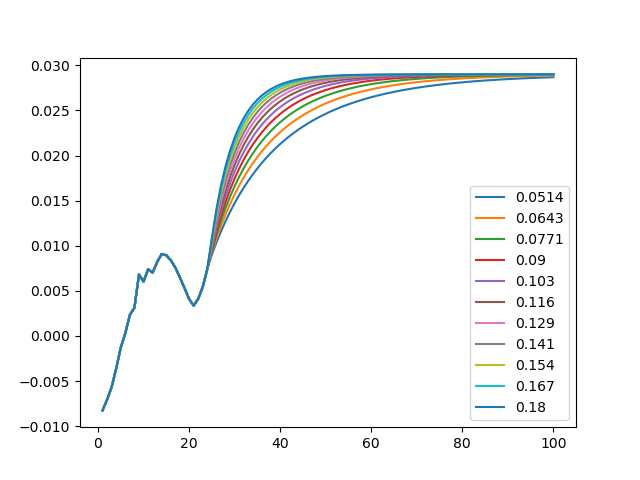# Smith-Wilson extrapolation with various alphas#

This notebook reads in the smithwilson model, and extrapolates forward rates using the Smith-Wilson method with various alphas, and draws the forward rates. See Project smithwilson for the details of the smithwilson model.

The source of the input data is Switzerland EIOPA spot rates with LLP 25 years available from the following source.

Source: RFR_spot_no_VA tab in EIOPA_RFR_20190531_Term_Structures.xlsx, archived in EIOPA_RFR_20190531.zip, avaialble on EIOPA’s Risk-Free Interest Rate Term Structures web site.

```import modelx as mx
import pandas as pd
```

The code below reads the `smithwilson` model from “model” folder, and assign `SmithWilson` space in the model to a global variable named `space`.

```space = mx.read_model("model").SmithWilson
```

The code below is for parametrizing the `SmithWilson` space by `x`. The parameter `x` takes integer values, and they can be positive and negative. the alpha in the `SmithWilson[x]` space is adjusted from the original alpha by `x` times 10%.

```def parametrize(x):
return {"refs": {"alpha": alpha * (1 + 0.1*x)}}

space.formula = parametrize
```

The code below creates the `ForwardRates` cells, which calculates extrapolated forward rates from the present values of bond prices.

```@mx.defcells
def ForwardRates(t):
return P(t) / P(t+1) - 1
```

The code below plots the extrapolated forward rates with various alphas.

```d = {"{0:.3}".format(space.alpha * (1 + 0.1*x)):
[space[x].ForwardRates[t] for t in range(1, 101)]
for x in range(-6, 5)}

df = pd.DataFrame(d, index=range(1, 101))

df.plot.line()
```Total running time of the script: ( 0 minutes 0.790 seconds)

Gallery generated by Sphinx-Gallery